# Preface

• This article was written for posting on the Numazu National College of Technology Advent Calendar 2018.
• For those who can do the basics of Processing, the explanation of the simple part is omitted.
• Due to the screen size, we recommend viewing on a PC.

Today is 12/14 ... That's right K </ font> Li </ font> S </ font> Ma </ font> font> Su </ font> (for the eve of the festival)

There are 10 days left. So, I'd like to prepare a Christmas card soon!

This time, I will explain using Processing, but I will also show you what I made using p5.js, which was my first challenge! Is it good to try new things (˘ω˘)

What is Processing? ?? (Simply speaking, a Java-based programming language for electronic art and design) ↓↓↓↓↓↓↓↓↓↓↓↓↓↓↓ Processing (official) https://processing.org/ Processing（Wiki） https://ja.wikipedia.org/wiki/Processing

Actually, the Arduino development environment you used in the technical college class is based on Processing. Similar to the Processing development environment.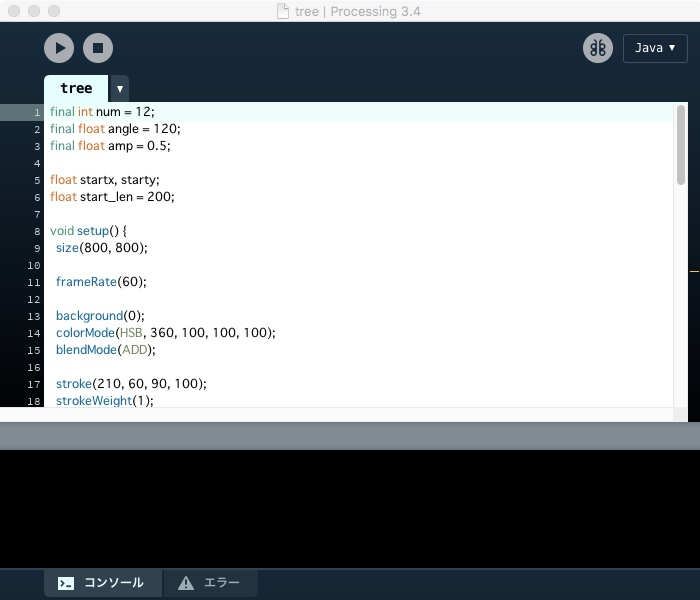You see, it's very similar!

# Chapter 1 The Christmas tree is an "ordinary tree".

First of all, Christmas is that, right?

It proliferated rapidly from the end of November and suddenly appeared indoors where trees should not have grown. A stationary weapon that supports the rear charge and damages the non-rear.

Well, it's a Christmas tree. (It ’s a really scary weapon ...)

But without this, Christmas won't happen, so be prepared.

so.... This is the tree I prepared.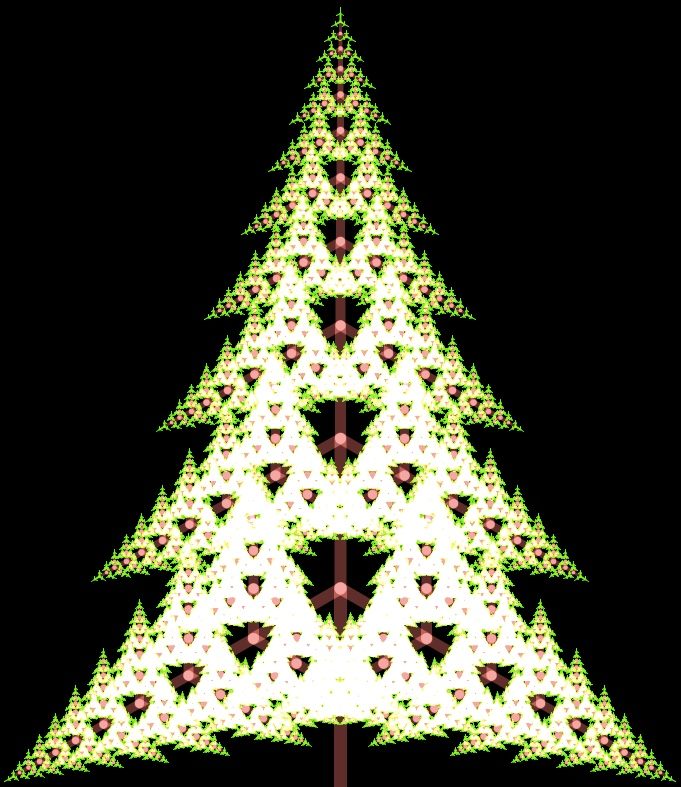It's a tree no matter how you look at it! It's a perfect Christmas tree that glows nicely! (pressure)

(Actually, the Christmas tree is a tree, so it's a well-known recursive function tree, isn't it? I tried my best, but even in the tree of this image, 3 ^ 12 = 531,441 times the function is called and calculated. If you do the drawing, the processing will be very heavy, so for the time being, I tried to make it look beautiful as it is a squishy tree ...)

That's why I decided to call this ordinary tree a Christmas tree (˘ω˘).

・ ・ ・ Hey, show me the code!

I understand, that feeling. I also found another person's work while studying Processing Eh ... what's going on with this? I don't know, I'm sorry! I think. If true, it would be better to think for yourself The recursive tree this time is famous, so I'll leave the source as it is.

### Christmas tree source code

Tree source code ← Click to open the source code

#### `tree.pde`

``````

final int num = 12;  //Number of recursion
final float angle = 120;  //Branch angle
final float amp = 0.5; //The length of the next branch is half that of the previous branch

float startx, starty;  //Initial position (root)
float start_len = 200; //First branch length

void setup() {
size(800, 800); //Screen size

frameRate(60); //60fps

background(0); //Make the background black
colorMode(HSB, 360, 100, 100, 100); //Use HSB instead of RGB
blendMode(ADD); //Change to the mode to issue as the colors overlap

stroke(210, 60, 90, 100); //Specify the line color

translate(width/2, height);//Move the origin of the coordinates to the center of the screen

startx=0; //Substitute the initial (tree root) position
starty=0; //Substitute the initial (tree root) position

tree(startx, starty, start_len, 0, 0); //Drawing a tree (recursive function)
}

void tree(float x, float y, float len, float stand, float step) {
float next_x, next_y; //Coordinates of the point at the tip of the next branch

next_x = x + len * sin(radians(stand)); //Calculate the tip coordinates of the next branch
next_y = y - len * cos(radians(stand)); //Calculate the tip coordinates of the next branch

strokeWeight(num+1-step); //Set the line thickness (becomes thinner toward the tip)

if (step<num-1) {
stroke(0, 60, 50, 80);  //Set the color of the branches
} else {
stroke(120, 100, 100, 100); //Set the color of the deepest branch of each recursion as a leaf
}
line(x, y, next_x, next_y); //Draw a branch

if (step!=num) { //Call the next function if the specified number of recursion has not been reached
tree(next_x, next_y, len * amp, stand - angle, step+1); //From the current branch-Calculate the next branch rotated 120 degrees
tree(next_x, next_y, len * amp * 1.5, stand, step+1);   //Calculate the next branch in the same orientation as the current branch
tree(next_x, next_y, len * amp, stand + angle, step+1); //Calculate the next branch rotated 120 degrees from the current branch
}
}
``````

To briefly explain what this program is doing, First, draw the first branch. From the tip of that branch, the next three branches are generated. From the three branches generated, three branches are generated for each. This is repeated the specified number of times.

You can see it in the image below.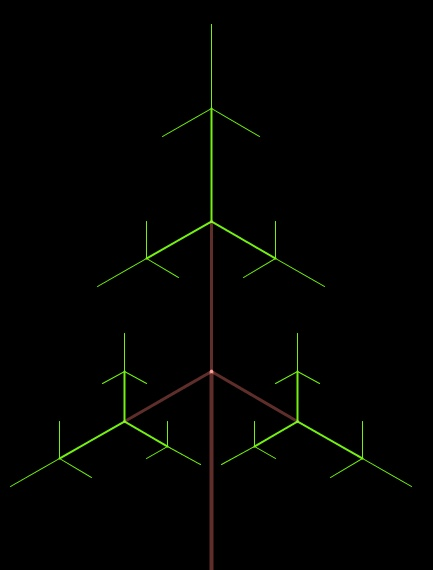This is what the tree looks like when the above process is performed three times. The start is the brown branch at the bottom center of the image. You can see that the branch is divided into three branches, and each of the three branches is further divided into three green branches.

Well, the explanation of the tree is easy I want snow next time!

# Chapter 2 Snowflakes are "ordinary trees" x 6.

Christmas is that! I thought I'd say Snowflakes have little to do with Christmas, right? What I noticed while writing this article ... (But I have already written the source code.)

It ’s just winter, so it ’s the same time as Christmas. It's pretty, I'm sure it's Christmas Must be a Christmas person! !!

So, when it comes to Christmas, this is it!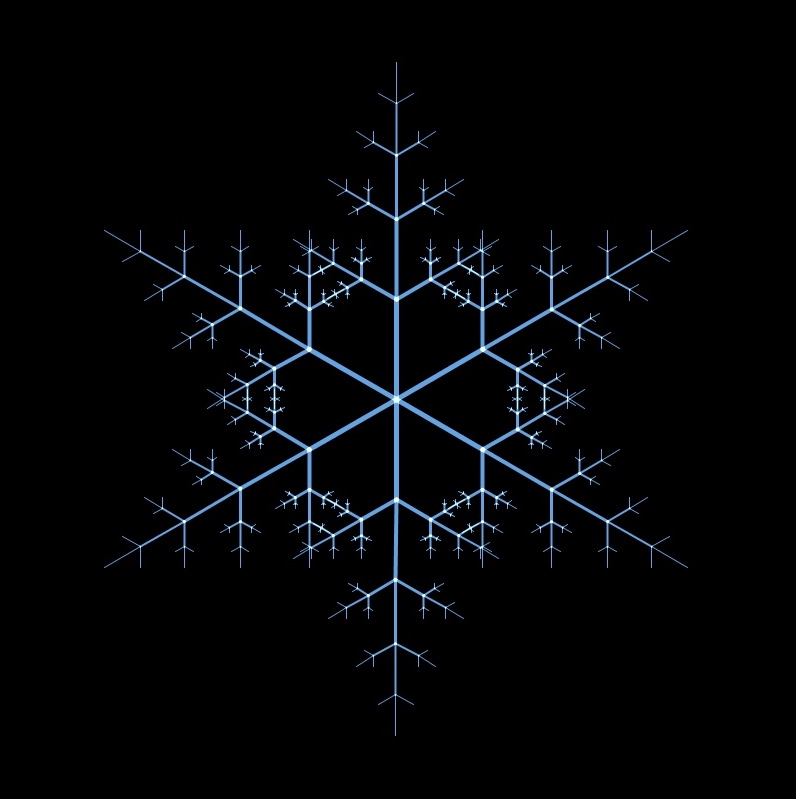If you have a good idea, you may have noticed. I'm using this snowflake and tree. Rather, I just prepared six rotated trees and changed the colors.

Snowflakes are made of Christmas trees. You were a great Christmas person!

So I will also put this source code here.

### Snowflake source code

Snowflake source code

#### `snow_crystal.pde`

``````
final int num=4;  //Number of recursion
final int corner=6; //Designation as a hexagonal crystal
final float angle=360/corner;//Angle to rotate the tree
final float amp=0.4;//Set the length of the next branch when drawing a tree

float startx, starty;//Initial position
float start_len=100;//Early branch length

void setup() {
size(800, 800);//Screen size

frameRate(60);//60fps

background(0);//Black background
colorMode(HSB, 360, 100, 100, 100);//Color uses HSB instead of RGB
blendMode(ADD);//Mode that emits light as the colors overlap

stroke(210, 60, 90, 100);//Crystal color

startx=0;//Initial position
starty=0;//Initial position
}

void draw() {
background(0); //Screen reset
translate(width/2,height/2);//Move the origin to the center of the screen
for(int i=0;i<corner;i++){   //Draw 6 trees.
tree(startx, starty, start_len, 0, 0);//Draw a tree.
rotate(2*PI/corner); //Rotate.
}

}

void tree(float x, float y, float len, float stand, float step) {
float next_x, next_y;

strokeWeight(num+1-step);
line(x, y, next_x, next_y);

if (step!=num) {
tree(next_x, next_y, len * amp, stand - angle, step+1);
tree(next_x, next_y, len * amp * 2, stand, step+1);
tree(next_x, next_y, len * amp, stand + angle, step+1);
}
}

``````

Well, the Christmas tree and snowflakes are ready After all, Christmas has a better atmosphere when it's snowing. So, let's make it snow next.

# Chapter 3 If all the falling snow is ellipse.

Gacky "I wish all the falling snow was Mel (Pee) Kiss." 2018Ver https://www.youtube.com/watch?v=Zq9zf41dh0o

I think so too If that happens, it seems that the outflow of population from Shizuoka will proceed in search of Mel (Pee) Kiss.

Unfortunately, there is no such thing in Processing. Instead, let's drop the "ellipse" used to draw the circle in Processing.

So ↓ ↓ ↓ here ↓ ↓ ↓ https://www.openprocessing.org/sketch/642846

This is the one I actually made. It snows when you open the URL. The left and right arrows on the keyboard blow the wind.For the time being, the snow in the back should be small and move slowly so that it looks 3D. I tried to express the snow in the foreground larger. I'll leave the source code for this as well.

### Snow source code

Snow source code

#### `snow_fall.pde`

``````

final float max_r=10; //Maximum radius of snow
final float min_r=1; //Minimum radius of snow
final float max_z=20; //Maximum depth
final float min_z=0; //Minimum depth
final float max_ys=3; //Maximum falling speed
final float min_ys=1; //Minimum falling speed
final int num=500; //Number of snow

float wind=0; //Wind strength
boolean flag=false; //Flag to prevent double key input

Snow[] snows=new Snow[num]; //Snow object array

void setup() {
fullScreen(); //Run in full screen
frameRate(60); //60fps
noCursor(); //Turn off the cursor

background(0); //The background is black
noSmooth(); //Turn off the function to draw a beautiful circle (for weight reduction)
noStroke(); //Do not use contour lines
fill(255,100); //Paint the snow in white

for (int i=0; i<num; i++) {
snows[i]=new Snow(i); //Snow generation
}
}

void draw() {
background(0);//Screen reset
for (int i=0; i<num; i++) {
snows[i].fall(wind); //Snow coordinate calculation
snows[i].show(); //Draw snow
}
}

class Snow{
float x;
float y;
float z;
float yspeed; //Snow falling speed
int my_num; //What number of snow was generated

Snow(int i){
x=random(-width/2-wind*100,width+width/2-wind*100); //Random initial coordinates
y=random(-height,0); //Random initial coordinates
z=random(min_z,max_z); //Random initial coordinates
r=map(z,min_z,max_z,min_r,max_r); //The radius is determined by the size of z
yspeed=map(z,min_z,max_z,min_ys,max_ys); //The fall speed is determined by the size of z
my_num = i; //Substitute your own generation number
}

void fall(float wind){
y=y+yspeed;  //The following coordinates are the current coordinates plus the fall speed

if(y>0){
x+=wind*map(z,min_z,max_z,0.3,1); //If the wind is blowing, the next coordinate will move in the X-axis direction
}

if(frameCount%20==0){
x+=random(-1.5,1.5); //The snow shakes a little
}

if(y>height){
snows[my_num]=new Snow(my_num); //When the snow reaches the ground, it will be regenerated.
}
}

void show(){
ellipse(x,y,r,r); //Draw a circle
}
}

void keyPressed(){ //Called automatically when a key is pressed

if(keyCode==RIGHT && flag==false && wind<10){ //When the right arrow is pressed
wind++; //Wind strength to the right+1
flag=true;
}else if(keyCode==LEFT && flag==false && wind>-10){ //When the left arrow is pressed
wind--;  //Wind strength to the left+1
flag=true;
}

}

void keyReleased(){
flag=false;
}

``````

The mechanism is simple. Generate 500 snow and calculate the next coordinates of 500 snow per frame. When it falls to the bottom of the screen, regenerate the snow and let it fall from the top of the screen. Just repeat this!

Well, if you get here Let's combine the Christmas tree, snowflakes, and snow into a card-like style.

# The final chapter is Merry Christmas.

Oops, I told you to make a Christmas card So far, the only thing that has a Christmas feeling is the Christmas tree. I have noticed.

Even if it looks like a Christmas card as it is, it does not feel like Christmas I mean, I don't have Santa? I think so. (I also think so)

But I think the important thing is that it says Merry Christmas With that, it must be Christmas.

So, in the end, it looks like this.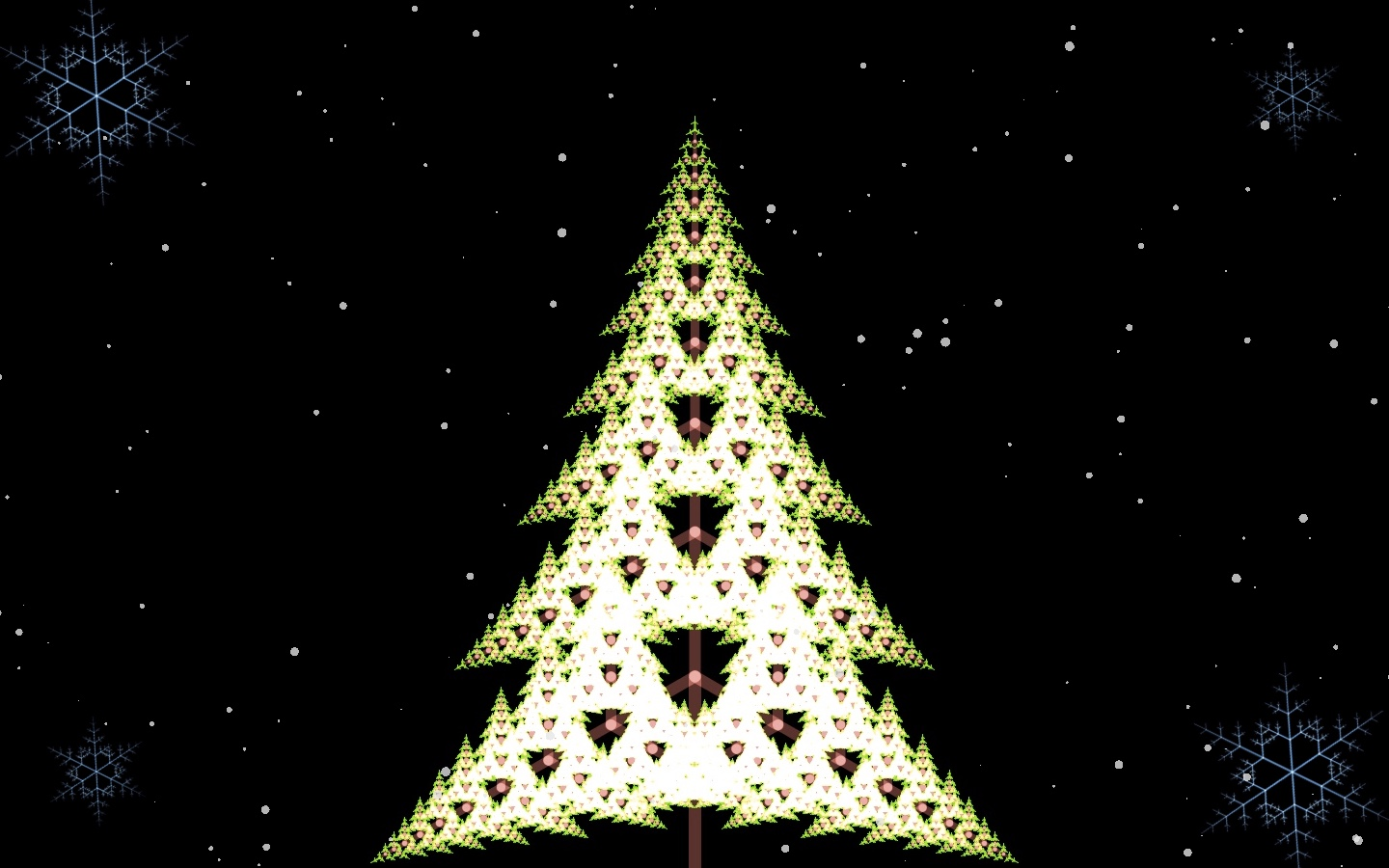Like that, when I wrote Merry Christmas, it became like Christmas!

↓ ↓ URL of the program that actually works ↓ ↓ https://www.openprocessing.org/sketch/643638

If you use a smartphone, the screen size is not enough, so open it on your PC. If you press Enter several times, a message will appear. Use the left and right arrow keys to blow the wind and move the snow.

The source code is here.

### Christmas card source code

Christmas card-like source code

#### `christmas.pde`

``````
final float max_r = 10;
final float min_r = 1;
final float max_z = 20;
final float min_z = 0;
final float max_ys = 3;
final float min_ys = 1;

PImage tree, crystal; //Variables for handling images

int num = 500;
float wind = 0;
boolean flag = false;

//animation
float x_L; //X coordinates of the two snowflakes on the left
float tree_x, tree_y; //Tree coordinates
int enter_count = 0; //Number of times the Enter key was pressed

float fade = 0; //Use with the effect that the characters gradually emerge
final float amp = 1.5; //Constants for adjusting the size of snowflakes

Snow[] snows = new Snow[num];

void setup() {
fullScreen(P2D); //Full screen mode,Processing becomes lighter by using P2D
frameRate(60);
noCursor();

background(0);
noSmooth();
noStroke();

textSize(50);
textAlign(CENTER);

for (int i=0; i<num; i++) {
snows[i] = new Snow(i);
}

tree_x = width/2-340; //The initial position of the tree 340 is the width of the image
tree_y = height-787; //The initial position of the tree 787 is the length of the image
x_L = 100; //Initial positions of the two snowflakes on the left
}

void draw() {
background(0);

image(tree, tree_x, tree_y); //Show tree

pushMatrix();//Save current axes//Snowflake on the upper left
translate(x_L, 100); //Move the origin
image(crystal, -100, -100, 200, 200); //Display snowflakes

pushMatrix();                      //Snowflake on the upper right
translate(width-100, 100);
image(crystal, -100, -100, 200, 200);
popMatrix();

pushMatrix();                      //Snowflake in the lower left
translate(x_L, height-100);
image(crystal, -100, -100, 200, 200);
popMatrix();

pushMatrix();                      //Snowflake in the lower right
translate(width-100, height-100);
image(crystal, -100, -100, 200, 200);
popMatrix();

fill(230, 200); //Snow color

for (int i=0; i<num; i++) {
snows[i].fall(wind);
snows[i].show();
}

Message(); //Function to display a message
}

void Message() {

if (enter_count==1) { //When the Enter key is pressed once
x_L = width/2; //Change the coordinates of the two snowflakes on the left
tree_x = width/10-340; //Change tree coordinates
} else if (enter_count==2) { //When the Enter key is pressed twice
fill(255, 0, 0, fade); //Text color
text("Merry Christmas!!", (width/2+width-100)/2, height*4/10); //Display message
} else if (enter_count==3) {
fill(255, 0, 0);
text("Merry Christmas!!", (width/2+width-100)/2, height*4/10);
text("Enjoy Processing", (width/2+width-100)/2, height*6/10);

} else if (enter_count==4) {
fill(255, 0, 0);
text("Merry Christmas!!", (width/2+width-100)/2, height*4/10);
fill(255, 0, 0);
text("Enjoy Processing", (width/2+width-100)/2, height*6/10);
textSize(30);
text("by Akiyoshi", (width/2+width-100)/2, height*8/10);
textSize(50);
}else if(enter_count==5){
fill(255, 0, 0);
text("Merry Christmas!!", (width/2+width-100)/2, height*4/10);
fill(255, 0, 0);
text("Enjoy Processing", (width/2+width-100)/2, height*6/10);
fill(255, 0, 0);
textSize(30);
text("by Akiyoshi", (width/2+width-100)/2, height*8/10);
textSize(50);
}else if(enter_count==6){
enter_count=0;  //Returns the count to 0 when the Enter key is pressed 6 times
x_L = 100; //Return the two snowflakes on the left to their initial positions
tree_x = width/2-340; //Reset the tree to its initial position
}
}

class Snow{
float x;
float y;
float z;
float r;
float yspeed;
int my_num;

Snow(int i){
x=random(-width/2-wind*100,width+width/2-wind*100);
y=random(-height,0);
z=random(min_z,max_z);
r=map(z,min_z,max_z,min_r,max_r);
yspeed=map(z,min_z,max_z,min_ys,max_ys);
my_num = i;
}

void fall(float wind){
y=y+yspeed;

if(y>0){
x+=wind*map(z,min_z,max_z,0.3,1);
}

if(frameCount%20==0){
x+=random(-1.5,1.5);
}

if(y>height){
snows[my_num] = new Snow(my_num);
}
}

void show(){
ellipse(x,y,r,r);
}
}

void keyPressed() {

if (keyCode==RIGHT && flag==false && wind<10) {
wind++;
flag=true;
} else if (keyCode==LEFT && flag==false && wind>-10) {
wind--;
flag=true;
}

if (keyCode==ENTER) { //Called when Enter is pressed
enter_count++; //Increase the number of times Enter is pressed by 1.
fade=0; //Return the text density to 0
}
}

void keyReleased() {
flag=false;
}
``````

It is like this. The mechanism is simple, just draw the Christmas tree. Simply change the size of the snowflake using scale () while rotating it frame by frame. For the falling snow, use the snowing program as it is. The characters gradually become darker, and the only thing that seems to appear is that the characters are changed from translucent to not transparent for each frame.

Read the source code, that? Are you using any images? ?? I think some people have noticed that.

That's right, I wrote a program to draw both a Christmas tree and a snowflake so far, but when I look at this source code, those two are images and the program that mainly uses snow only.

There is a reason for this. To summarize briefly, the amount of calculation for Christmas trees and snowflakes is amazing. If you move them all together, the processing will be heavy, and the falling snow will become squishy! In the first place, the tree and the snowflake do not move in particular, so is it too wasteful to recalculate to draw each frame? ??

So, this time I made an image of the tree and snowflake I made. If you really don't like it, I think there is nothing you can't do if you have a pretty good computer.

However, I think it's good for beginners in Processing because it will be a practice to handle images.

# the end

With this kind of feeling, a Christmas card-like thing was made. Wouldn't it be a card if this was further imaged and printed? If you port it to p5.js, upload it to Open Processing, and give the URL to your friends, you can give it as a working Christmas card!

I usually write the source code appropriately and make interesting things that were born by chance. It was hard to make something like a Christmas tree or snow (˘ω˘)

By the way, if you play with the source code you made this time as usual The Christmas tree looks like this.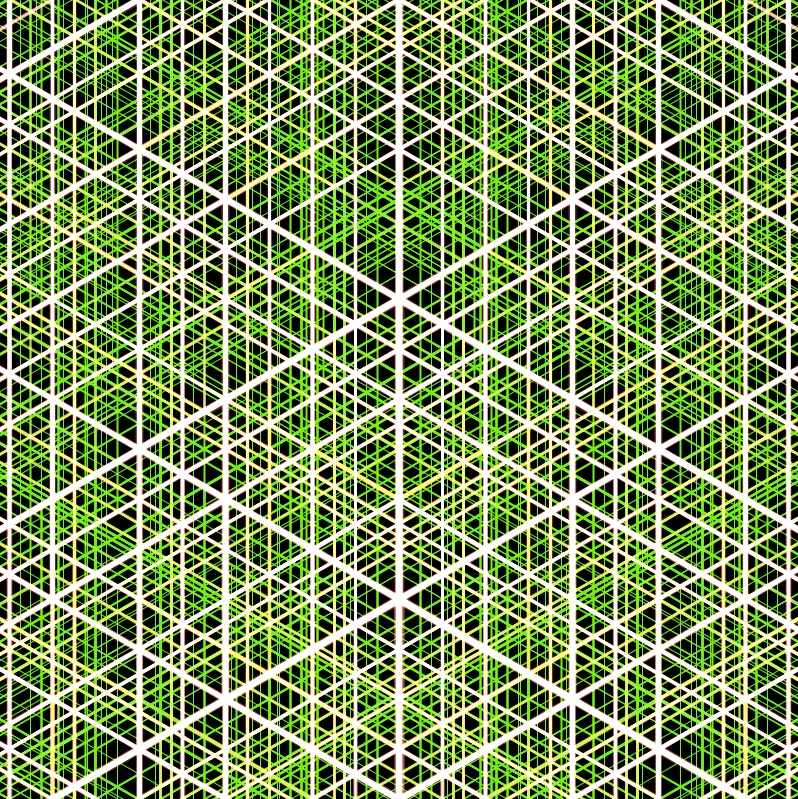The snowflake looks like this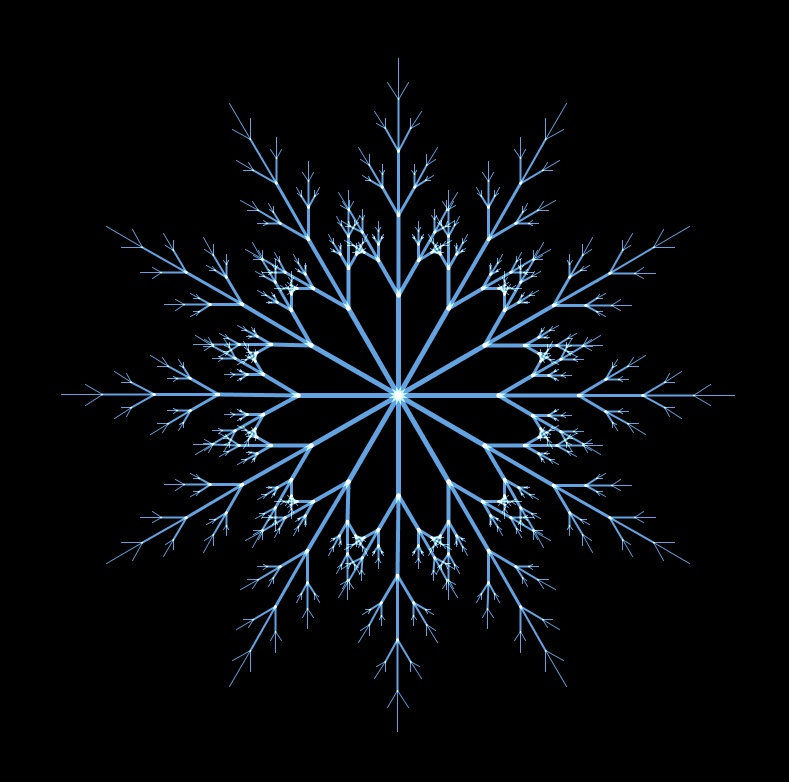Furthermore, it will be like this.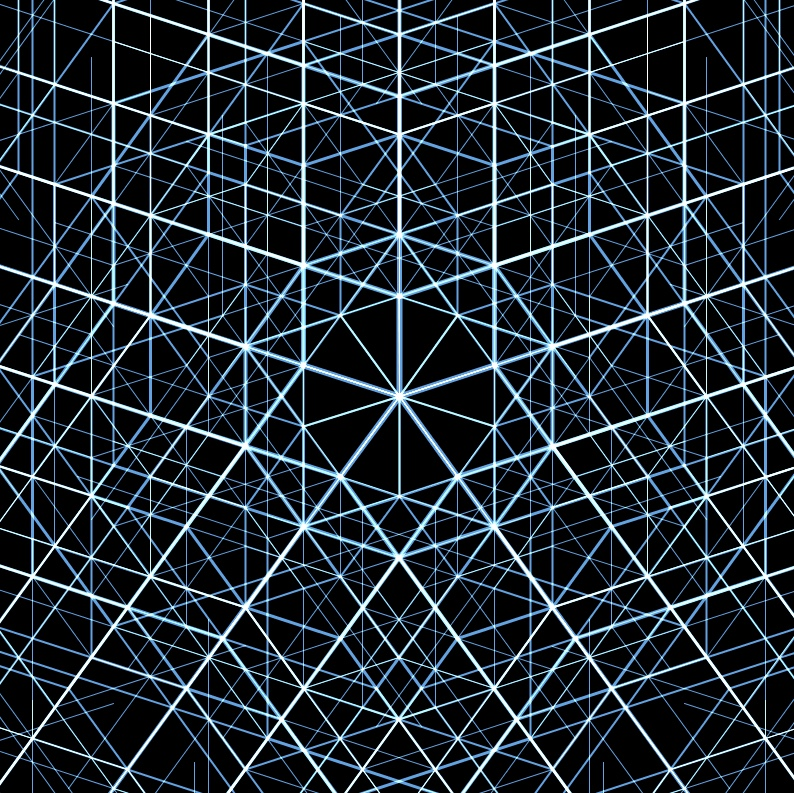After all, it's fun to play with the source code and make something that looks interesting! !! Everyone, please try Processing!